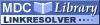Search
Browse
Statistics
Feeds

A complete enumeration and classification of two-locus disease modelsItem Type: Article A complete enumeration and classification of two-locus disease models Li, W.T. and Reich, J. There are 512 two-locus, two-allele, two-phenotype, fully penetrant disease models. Using the permutation between two alleles, between two loci, and between being affected and unaffected, one model can be considered to be equivalent to another model under the corresponding permutation. These permutations greatly reduce the number of two-locus models in the analysis of complex diseases. This paper determines the number of nonredundant two-locus models (which can be 102, 100, 96, 51, 50, or 58, depending on which permutations are used, and depending on whether zero-locus and single-locus models are excluded). Whenever possible, these nonredundant two-locus models are classified by their property. Besides the familiar features of multiplicative models (logical AND), heterogeneity models (logical OR), and threshold models, new classifications are added or expanded: modifying-effect models, logical XOR models, interference and negative interference models (neither dominant nor recessive), conditionally dominant/recessive models, missing lethal genotype models, and highly symmetric models. The following aspects of two-locus models are studied: the marginal penetrance tables at both loci, the expected joint identity-by-descent (IBD) probabilities, and the correlation between marginal IBD probabilities at the two loci. These studies are useful for linkage analyses using single-locus models while the underlying disease model is two-locus, and for correlation analyses using the linkage signals at different locations obtained by a single-locus model. Correlation, Epistasis, Identity by Descent, Two-locus Model, Animals, Drosophila Human Heredity 0001-5652 Karger (Switzerland) 50 6 334-349 1 November 2000 https://doi.org/10.1159/000022939 View item in PubMed

Repository Staff Only: item control page

Open Access
MDC Library